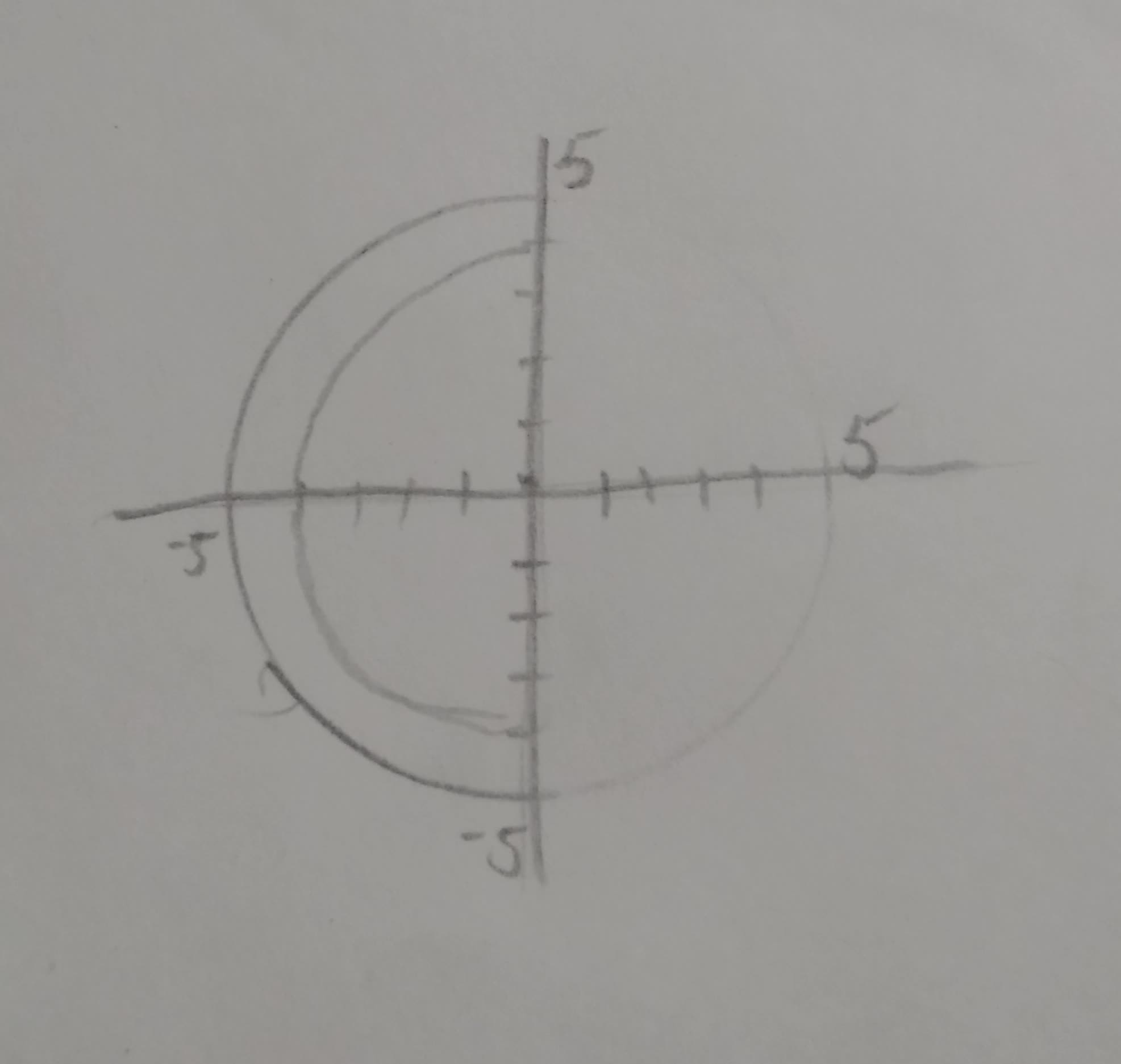# For the region R below, write \int \int_Z f dAbabeeb0oL 2021-09-11 Answered

For the region R below, write $\int {\int }_{Z}fdA$ as an integral in polar coordinates.Use t for $\theta$ in your expressions.

You can still ask an expert for help

• Questions are typically answered in as fast as 30 minutes

Solve your problem for the price of one coffee

• Math expert for every subject
• Pay only if we can solve itestenutC
From the given figure, it is seen that the region R is bounded by two circles, ${x}^{2}+{y}^{2}=9\phantom{\rule{1em}{0ex}}\text{and}\phantom{\rule{1em}{0ex}}{x}^{2}+{y}^{2}=16$
Therefore, region R can be defined as
$R=\left\{{\left(r,\theta \right)}^{3}\le r\le 4,0\le \theta \le \pi \right\}$
it is known that,
$\int {\int }_{R}fdA={\int }_{{\theta }_{1}}^{{\theta }_{2}}f\left(r\mathrm{cos}\theta ,r\mathrm{sin}\theta \right)rdrd\theta$
Therefore, in polar co-ordinate,
$\int {\int }_{R}fdA={\int }_{0}^{\pi }{\int }_{3}^{4}f\left(r\mathrm{cos}\theta ,r\mathrm{sin}\theta \right)rdrd\theta$ (i)
Thus, comparing (i) with $\int {\int }_{R}fdA={\int }_{0}^{\pi }{\int }_{3}^{4}f\left(r\mathrm{cos}\theta ,r\mathrm{sin}\theta \right)rdrd\theta$ it is inferred that
a = 0
$b=\pi$
c = 3
d = 4
With $dA=rdrd\theta$